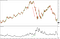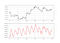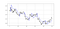# The SuperTrend Indicator in Python — Coding and Back-Testing Its Strategy

## How to code it, back-test it, and evaluate it on FX Trading

This rare gem is a trend-following indicator that can be used either as a trading system or as a way to place your stops. We will introduce the intuition of the SuperTrend indicator, code it in Python, back-test a few strategies, and present our conclusion. Of course, just like any other indicator, the SuperTrend can find its place within a bigger trading system but not take over the whole decision-making process.

# Building Blocks

The first concept we should understand before creating the SuperTrend indicator is volatility. We sometimes measure volatility using the Average True Range. Although the ATR is considered a lagging indicator, it gives some insights as to where volatility is right now and where has it been last period (day, week, month, etc.). But before that, we should understand how the True Range is calculated (the ATR is just the average of that calculation).

The true range is simply the greatest of the three price differences:

• High - Low

Once we have got the maximum out of the above three, we simply take an average of n periods of the true ranges to get the Average True Range. Generally, since in periods of panic and price depreciation we see volatility go up, the ATR will most likely trend higher during these periods, similarly in times of steady uptrends or downtrends, the ATR will tend to go lower. One should always remember that this indicator is very lagging and therefore has to be used with extreme caution. Below is the function code that calculates a form of the ATR.

`def atr(Data, atr_lookback, high, low, close, whereTR, whereATR):        # TR    for i in range(len(Data)):                    Data[i, whereTR] = max(Data[i, high] - Data[i, low],                    abs(Data[i, high] - Data[i - 1, close]),                    abs(Data[i, low] - Data[i - 1, close]))            Data[0, whereATR] = Data[0, high] - Data[0, low]            Data[atr_lookback - 1, whereATR] = Data[0:atr_lookback - 1,  whereTR].mean()        first_atr = Data[0, whereATR]        for i in range(len(Data)):                Data[0, whereATR] = first_atr                 Data[i, whereATR] = (((Data[i - 1, whereATR] * (atr_lookback - 1)) + Data[i, whereTR]) / atr_lookback)        return Data`

The below chart shows the EURUSD daily values versus the 14-Day ATR. Notice the slightly negative visual correlation between the two. Also, we notice the spike in the ATR when the EURUSD started plummeting during the 2008 financial crisis.The ATR goes up whenever recent volatility is increasing and thus providing confirmation. (Image by Author)

Now that we have understood what the ATR is and how to calculate it, we can proceed further with the SuperTrend indicator. But wait, what if we try something more exciting? What if we try to make the ATR less lagging and consequently improve the SuperTrend’s efficiency? By using a simple manipulation, we can create a more dynamic Average True Range, here is the idea followed by the Python code:

The Average True Range is calculated using a simple moving average. We want to try out an ATR that uses an exponential moving average. This new modified ATR which we will call eATR (short for Exponential ATR) will have more reactions to recent volatility and be able to capture it better. Since the SuperTrend uses the ATR as a variable in its formula, it will surely change it from the common SuperTrend. We can call this new indicator eSuperTrend for the remainder of the article so that it will be easier to refer to it.

`def eATR(Data, atr_lookback, high, low, close, whereTR):  # TR for i in range(len(Data)):  try:    Data[i, whereTR] = max(Data[i, high] — Data[i, low],   abs(Data[i, high] — Data[i — 1, close]),   abs(Data[i, low] — Data[i — 1, close]))   except ValueError:   pass Data[0, whereTR] = 0  Data = ema(Data, 2, atr_lookback, whereTR, whereTR + 1, whereTR + 2) return Data`

Since it is out of scope of this article, if you would like to see how to code the ema (exponential moving average) function, it is described in the article below. Here is an example of the EURUSD chart with the eATR(10). This means that the eATR is calculated based on the last 10 values.EURUSD in the first panel with eATR in the second panel. (Image by Author)

# Creating The Function

Now that we have our plan of attack laid out in front of us, we can proceed by understanding the SuperTrend and coding it, and finally back-testing it.

1. Calculate the eATR using the function provided above.

The indicator seeks to provide entry and exit levels for trend followers. You can think of it as a moving average or an MACD. Its uniqueness is its main advantage and although its calculation method is much more complicated than the other two indicators, it is intuitive in nature and not that hard to understand. Basically, we have two variables to choose from. The eATR lookback and the multiplier’s value. The former is just the period used to calculated the eATR while the latter is generally an integer (usually 2 or 3).

The first thing to do is to average the high and low of the price bar, then we will either add or subtract the average with the selected multiplier multiplied by the eATR as shown in the above formulas. This will give us two arrays, the basic upper band and the basic lower band which form the first building blocks in the SuperTrend indicator. The next step is to calculate the final upper band and the final lower band using the below formulas.

It may seem complicated but most of these conditions are repetitive and in any case, I will provide the Python code below so that you can play with the function and optimize it to your trading preferences. Finally, using the previous two calculations, we can find the SuperTrend or in our case the eSuperTrend (as we are using the eATR to calculate it as opposed to the normal ATR).

`def supertrend(Data, multiplier, lookback):        for i in range(len(Data)):                    # Average Price            Data[i, 5] = (Data[i, 1] + Data[i, 2]) / 2            # Basic Upper Band            Data[i, 6] = Data[i, 5] + (multiplier * Data[i, 4])            # Lower Upper Band            Data[i, 7] = Data[i, 5] - (multiplier * Data[i, 4])        # Final Upper Band    for i in range(len(Data)):                if i == 0:            Data[i, 8] = 0                    else:              if (Data[i, 6] < Data[i - 1, 8]) or (Data[i - 1, 3] > Data[i - 1, 8]):                Data[i, 8] = Data[i, 6]                        else:                Data[i, 8] = Data[i - 1, 8]        # Final Lower Band    for i in range(len(Data)):                if i == 0:            Data[i, 9] = 0                    else:              if (Data[i, 7] > Data[i - 1, 9]) or (Data[i - 1, 3] < Data[i - 1, 9]):                Data[i, 9] = Data[i, 7]                        else:                Data[i, 9] = Data[i - 1, 9]          # SuperTrend    for i in range(len(Data)):                if i == 0:            Data[i, 10] = 0                elif (Data[i - 1, 10] == Data[i - 1, 8]) and (Data[i, 3] <= Data[i, 8]):            Data[i, 10] = Data[i, 8]                elif (Data[i - 1, 10] == Data[i - 1, 8]) and (Data[i, 3] > Data[i, 8]):            Data[i, 10] = Data[i, 9]                elif (Data[i - 1, 10] == Data[i - 1, 9]) and (Data[i, 3] >= Data[i, 9]):            Data[i, 10] = Data[i, 9]                elif (Data[i - 1, 10] == Data[i - 1, 9]) and (Data[i, 3] < Data[i, 9]):            Data[i, 10] = Data[i, 8]                return Data`

Applying the above function on an OHLC array will give us something like the below when we plot it. The way we should understand the indicator is that when it goes above the market price, we should be looking to short and when it goes below the market price, we should be looking to go long as we anticipate a bullish trend. Remember that the SuperTrend is a trend-following indicator. The aim here is to capture trends at the beginning and to close out when they are over.Example of the eSuperTrend indicator on USDCHF pair. (Image by Author)

If you are interested in trend-following indicators, then this article on moving averages might interest you:

# Back-Testing

Now, it is time to back-test the eSuperTrend. Surely, a sole indicator is not enough for a full trading strategy and hence we are looking for added-value when we back-test indicators and that can be reflected by an average tilt to the upside in the equity curves. This means that to judge an indicator, it does not have to be a hugely profitable one because we know that it will not be used alone in trading and thus, we are looking for a contribution factor. We can also use the signal charts to subjectively judge the quality of the signals. The conditions of the eSuperTrend are fairly easy:

• When the indicator drops below the market price, we initiate a long order and hold it until we have a short signal.

Below is a summary of the equity curves using the above conditions on an H3 time frame since 2000 on 10 major currency pairs.

# Conclusion

We have seen together this powerful indicator and learnt how to code it and now it is time to evaluate it. Broadly speaking, it looks acceptable on some pairs and much more optimization can be done with the addition of other indicators and techniques. Also, the risk management method used in the back-test was very basic and more complicated techniques can be used. My personal experience is that this indicator shows some potential and can be improved.

Written by

Written by

## Sofien Kaabar

#### Institutional FOREX Strategist | Trader | Data Science Enthusiast. Author of the Book of Back-tests: https://www.amazon.com/dp/B089CWQWF8